CAT  >  Maths and Logical Reasoning Test- 2

# Maths and Logical Reasoning Test- 2

Test Description

## 15 Questions MCQ Test TISS-NET Section Wise & Full Mock Test Series | Maths and Logical Reasoning Test- 2

Maths and Logical Reasoning Test- 2 for CAT 2022 is part of TISS-NET Section Wise & Full Mock Test Series preparation. The Maths and Logical Reasoning Test- 2 questions and answers have been prepared according to the CAT exam syllabus.The Maths and Logical Reasoning Test- 2 MCQs are made for CAT 2022 Exam. Find important definitions, questions, notes, meanings, examples, exercises, MCQs and online tests for Maths and Logical Reasoning Test- 2 below.
Solutions of Maths and Logical Reasoning Test- 2 questions in English are available as part of our TISS-NET Section Wise & Full Mock Test Series for CAT & Maths and Logical Reasoning Test- 2 solutions in Hindi for TISS-NET Section Wise & Full Mock Test Series course. Download more important topics, notes, lectures and mock test series for CAT Exam by signing up for free. Attempt Maths and Logical Reasoning Test- 2 | 15 questions in 15 minutes | Mock test for CAT preparation | Free important questions MCQ to study TISS-NET Section Wise & Full Mock Test Series for CAT Exam | Download free PDF with solutions
 1 Crore+ students have signed up on EduRev. Have you?
Maths and Logical Reasoning Test- 2 - Question 1

### A student obtained 87 average marks in 8 subjects. If the students highest gaining subject is 2 marks more than the second-ranked subject. If these two subjects are eliminated then the average of the remaining subjects will become 85. The highest marked subject is:

Detailed Solution for Maths and Logical Reasoning Test- 2 - Question 1

Let the highest marks obtained by the student be x

Then, second-highest marks x = 2

Sum of marks of these 2 subjects = (87 x 8) - (85 x 6)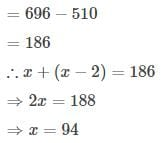Hence, the correct option is (C).

Maths and Logical Reasoning Test- 2 - Question 2

### What is the value of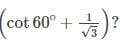Detailed Solution for Maths and Logical Reasoning Test- 2 - Question 2

Given: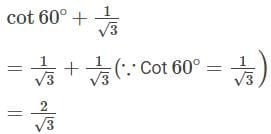Hence, the correct option is (C).

Maths and Logical Reasoning Test- 2 - Question 3

### Three persons walking together in the morning. The distance of their one footstep is 80 cm, 85 cm, and 90 cm respectively. How much minimum distance they cover so that distance can be measured with the complete number (integer number) of their footsteps completely?

Detailed Solution for Maths and Logical Reasoning Test- 2 - Question 3

Given: Their steps measure 80 cm, 85 cm, and 90 cm.

We have to find the L.C.M. of the measures of their steps to calculate the required distance each should walk.

L.C.M. of 80 cm, 85 cm, and 90 cm.

80=24×5

85=17×5

90=2×32×5

L.C.M 80,85,90=24×32×5×17 =12240 cm

So, the minimum distance each should walk so that all can cover the same distance in complete steps are 122.40 m.

Hence, the correct option is (A).

Maths and Logical Reasoning Test- 2 - Question 4

A shopkeeper sells rice on 10% profit and also uses 20% less weight, his total percent of profit is:

Detailed Solution for Maths and Logical Reasoning Test- 2 - Question 4

Let's take cost Price =100

Then, Selling Price =100+10% of 100=100+10=110

Since we get 1 kg amount with fair weights.

⇒ With unfair weights, we will get 20% less

=1000-20% of 1000=800 g =0.8 kg

Now, For 0.8 kg ⇒S.P.=110 Rs.

1 kg ⇒S.P.=110/0.8=137.5 Rs.

Net profit =137.5-100=37.5 Rs.

Net Profit %=(37.5/100) x 100=37.5%

Hence, the correct option is (D).

Maths and Logical Reasoning Test- 2 - Question 5

Direction: What will come in place of the question mark (?) in the following number series?

8,24,12,36,18,54,?

Detailed Solution for Maths and Logical Reasoning Test- 2 - Question 5

The pattern is-

8 × 3 = 24

24 ÷ 2 = 12

12 × 3 = 36

36 ÷ 2 = 18

18 × 3 = 54

54 ÷ 2 = 27

Hence, the correct option is (A).

Maths and Logical Reasoning Test- 2 - Question 6

if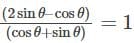then the value of cot θ is:

Detailed Solution for Maths and Logical Reasoning Test- 2 - Question 6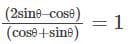Dividing by sinθ in the numerator and denominator,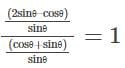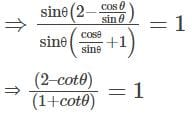⇒ 2 - cot θ = 1 + cot θ

⇒ 2 cot θ = 1

⇒ cot θ = ½

Hence, the correct option is (A).

Maths and Logical Reasoning Test- 2 - Question 7

In a certain code language, '×' represents '+', '÷' represents '×', '-' represents '÷' and '+' represents '-'. Find out the answer to the following question.

42 + 36 – 6 ÷ 15 ×​ 8 ÷ 20 + 20 – 5 = ?

Detailed Solution for Maths and Logical Reasoning Test- 2 - Question 7

Given expression:

42 + 36 – 6 ÷ 15 x 8 ÷ 20 + 20 – 5 = ?

After changing the symbols:

42 – 36 ÷ 6 × 15 + 8 x 20 – 20 ÷ 5

= 42 – 6 x 15 + 160 – 4

= 42 – 90 + 160 – 4

= 108

Hence, the correct option is (B).

Maths and Logical Reasoning Test- 2 - Question 8

A series is given with one term missing. Select the correct alternative from the given ones that will complete the series.

EJK, DIJ, BGH, ?

Detailed Solution for Maths and Logical Reasoning Test- 2 - Question 8

The pattern follows here is:-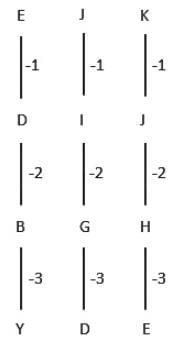Hence, the correct option is (A).

Maths and Logical Reasoning Test- 2 - Question 9

Four pairs of number have been given, out of which three are alike in some manner, while one is different. Choose out the odd one.

Detailed Solution for Maths and Logical Reasoning Test- 2 - Question 9

The pattern followed here is:

The addition is two numbers is a multiple of 10.

Option (A) → 23 - 18 → 23 + 18 = 41, Not divisible by 10.

Option (B) → 30 - 20 → 30 + 20 = 50 → 10 x 5 = 50

Option (C) → 62 - 8 → 62 + 8 = 70 → 10 x 7 = 70

Option (D) → 21 - 9 → 21 + 9 = 30 → 10 x 3 = 30

All except, '23 - 18' follow the same pattern.

Hence, the correct option is (A).

Maths and Logical Reasoning Test- 2 - Question 10

Calculate the distance (in cm) of the chord from the centre of the circle, if the chord of length 48 cm is drawn in the circle of radius 25 cm.

Detailed Solution for Maths and Logical Reasoning Test- 2 - Question 10

The given figure can be drawn as,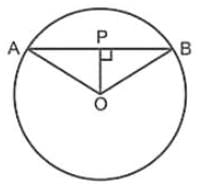Let O be the centre of the circle, AB be the chord and P is the centre point of chord AB, such that OP is the distance of the chord from the centre of the circle

Now, OA = OB = radius of circle = 25 cm

Length of chord = AB = 48 cm

⇒ PB = AB/2 = 48/2 = 24 cm

Applying Pythagoras theorem in ΔOPB,

⇒ OB2 = OP2 + PB2

⇒ 252 = OP2 + 242

⇒ OP2 = 625 - 576 = 49

⇒ OP = √49 = 7 cm

∴ Distance of chord from centre of circle = 7 cm

Hence, the correct option is (A).

Maths and Logical Reasoning Test- 2 - Question 11

Direction: In the question below there are three statements followed by three conclusions I, II and III. You have to take the three given statements to be true even if they seem to be at variance from commonly known facts and then decide which of the given conclusions logically follows from the given statements disregarding commonly known facts.

Statements:

I. Some cats are dogs.

II. All dogs are monkeys.

III. All monkeys are pigs.

Conclusions:

I. Some cats are monkeys.

II. No pig is cat.

III. All dogs are pigs.

Detailed Solution for Maths and Logical Reasoning Test- 2 - Question 11

The least possible Venn diagram is given below,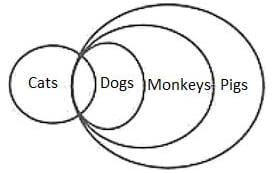Conclusions:

I. Some cats are monkeys → True (Some cats are dogs and all dogs are monkeys, so some cats are monkeys.)

II. No pig is cat → False (Some cats are dogs, all dogs are monkeys and all monkeys are pigs, so some cats are pigs.)

III. All dogs are pigs → True (All dogs are monkeys and all monkeys are pigs, so all dogs are pigs.)

So, only conclusion I and III follow.

Hence, the correct option is (A).

Maths and Logical Reasoning Test- 2 - Question 12

Select the option that is related to the third term in the same way as the second term is related to the first term.

Pitch : Cricket :: Arena : ?

Detailed Solution for Maths and Logical Reasoning Test- 2 - Question 12

Pitch is the place where cricket is played.

Similarly, arena is the place where Wrestling is done.

Hence, the correct option is (C).

Maths and Logical Reasoning Test- 2 - Question 13

If tan4θ + tan2θ = 1, then the value of cos4θ + cos2θ is:

Detailed Solution for Maths and Logical Reasoning Test- 2 - Question 13

Given: tan4θ + tan2θ = 1

⇒tan2θ (tan2θ + 1)

⇒ tan2θ. sec2θ = 1 {∵ sec2θ = 1 + tan2θ}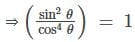⇒ 1– cos2θ = cos4 θ {∵ sin2 θ = 1 – cos2θ}

⇒ cos4 θ + cos2 θ = 1

Hence, the correct option is (C).

Maths and Logical Reasoning Test- 2 - Question 14

In ΔABC, ∠ABC = 70°, ∠BCA = 40°. O is the point of intersection of the perpendicular bisectors of the sides, then the angle ∠BOC is

Detailed Solution for Maths and Logical Reasoning Test- 2 - Question 14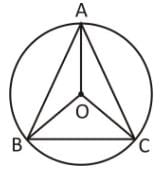OA = OB = OC = Circum-radius

In ΔABC, we know that

∠ABC + ∠BCA + ∠BAC = 180°

∠BAC = 180° – 70° – 40° = 70°

We know that, The angle subtended by an arc of a circle at the centre is double the angle subtended by it at any point on the remaining part of the circle.

∴ ∠BOC = 2 x ∠BAC

= 2 x 70° = 140°

Hence, the correct option is (D).

Maths and Logical Reasoning Test- 2 - Question 15

if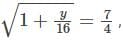then find the value of 5y – 15.

Detailed Solution for Maths and Logical Reasoning Test- 2 - Question 15

Given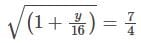Squaring both sides,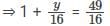⇒ 16 + y = 49

⇒ y = 49 – 16 = 33

∴ 5y – 15 = 5 x (33) – 15

= 165 – 15 = 150

Hence, the correct option is (C).

## TISS-NET Section Wise & Full Mock Test Series

28 tests
 Use Code STAYHOME200 and get INR 200 additional OFF Use Coupon Code
Information about Maths and Logical Reasoning Test- 2 Page
In this test you can find the Exam questions for Maths and Logical Reasoning Test- 2 solved & explained in the simplest way possible. Besides giving Questions and answers for Maths and Logical Reasoning Test- 2, EduRev gives you an ample number of Online tests for practice

## TISS-NET Section Wise & Full Mock Test Series

28 tests

### How to Prepare for CAT

Read our guide to prepare for CAT which is created by Toppers & the best Teachers